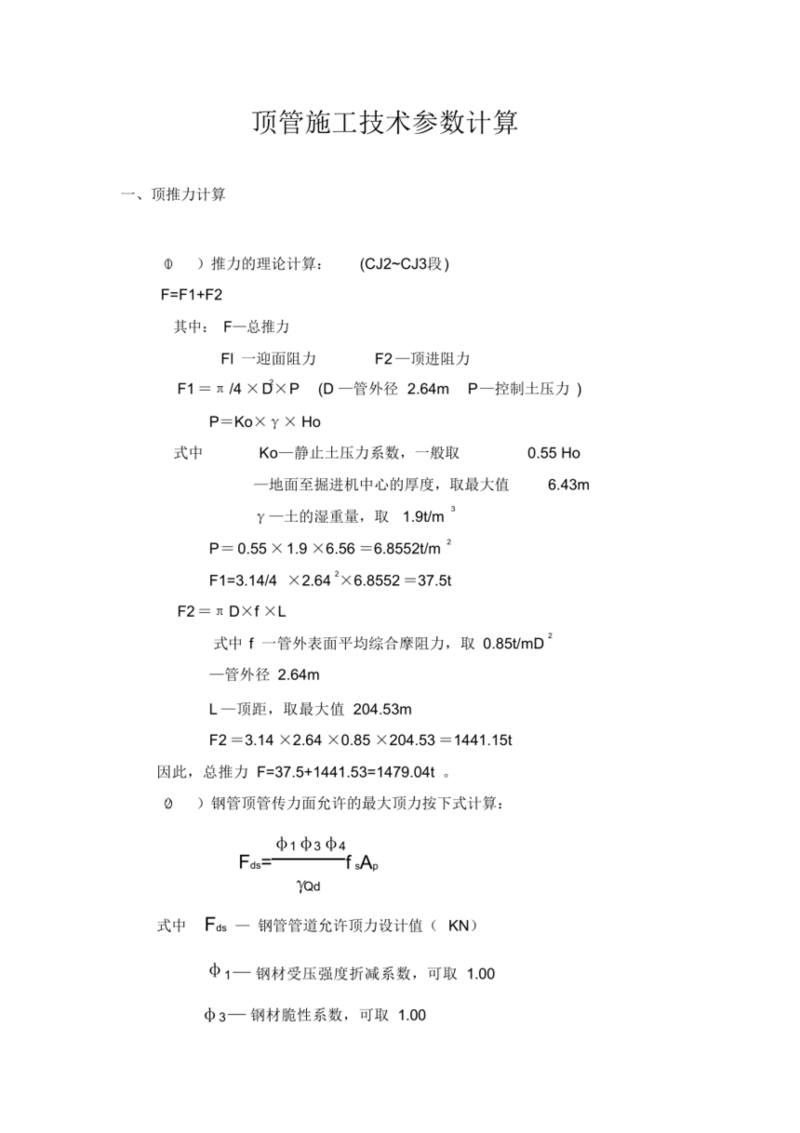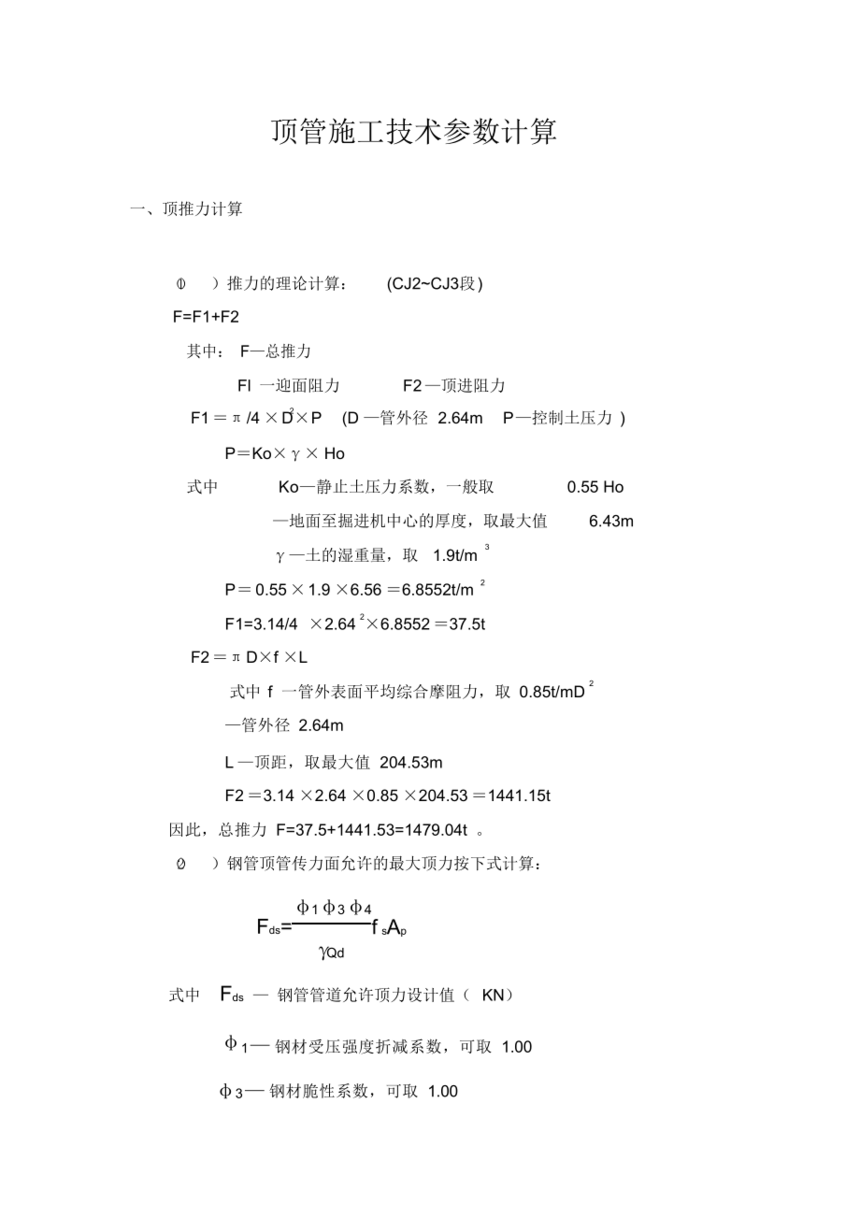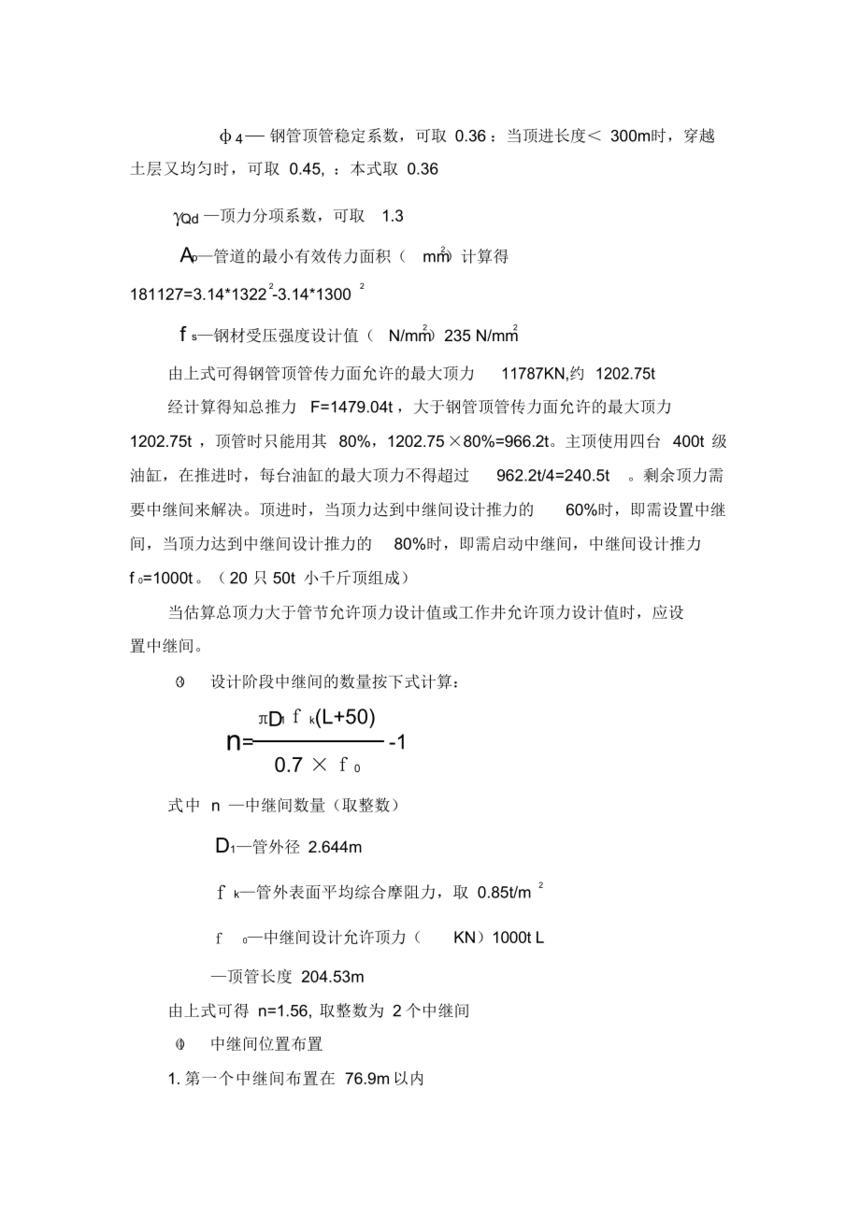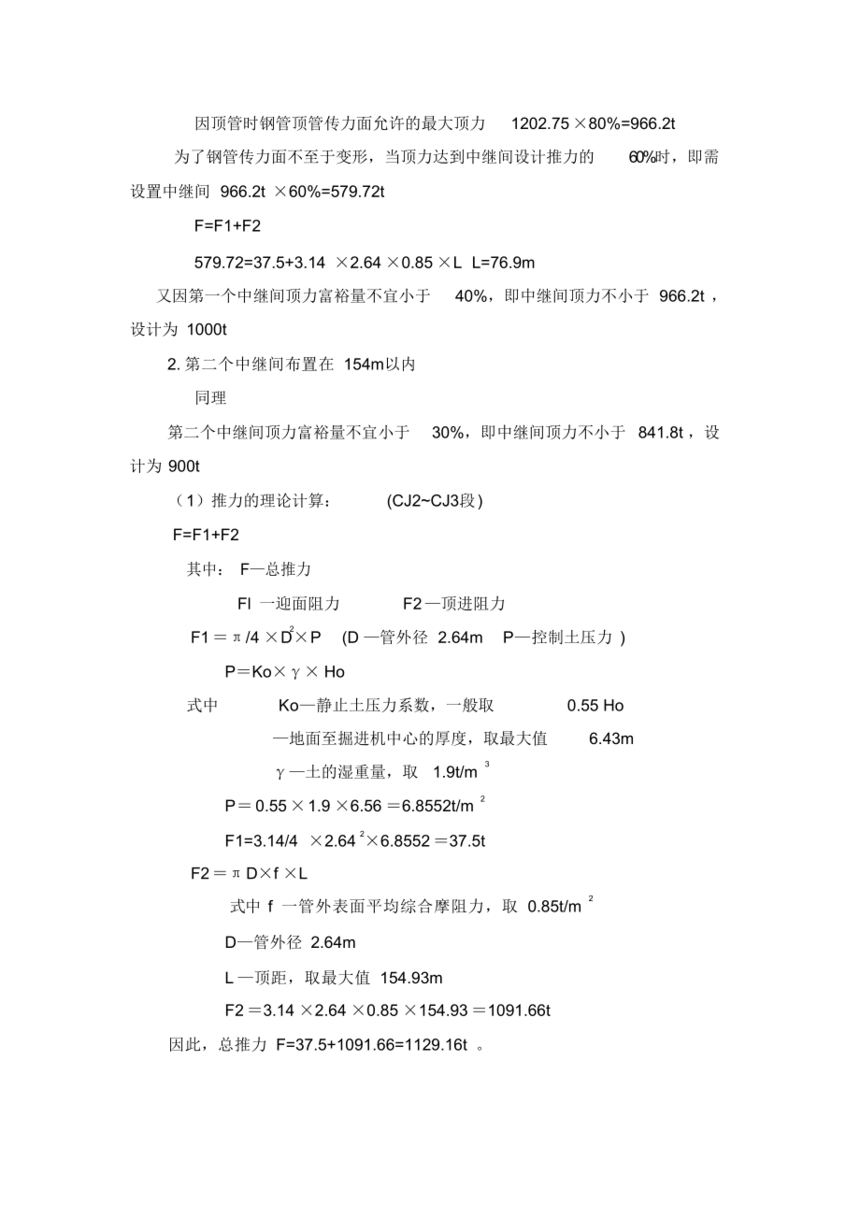# 南充远锦市政工程有限公司

 首页 >> 资讯 >>南充水泥制品资讯 >> 南充水泥制品顶管施工技术参数计算

# 南充水泥制品顶管施工技术参数计算•)推力的理论计算:(CJ2~CJ3段)F=F1+F2

FlI一迎面阻力

F2—顶进阻力

F1 =T /4 ×D×P(D—管外径2.64mP一控制土压力)

P=Ko×y × Ho

Ko—静止土压力系数，一般取

0.55 Ho

—地面到掘进机中点的厚度，取***值

6.43m

—土的湿重量，取1.9t/m '

=0.55×1.9×6.56=6.8552t/m ?F1=3.14/4 ×2.642×6.8552=37.5tF2=开 D×f×L

L—顶距，取***值204.53m

F2=3.14×2.64×0.85×204.53=1441.15t因此，总推力F=37.5+1441.53=1479.04t 。

）钢管顶管传力面允许的***顶力按下式计算:

13 4

Fds=-

1—钢材受压强度折减系数，可取1.003—钢材脆性系数，可取1.00

4—钢管顶管稳定系数，可取0.36:当顶进长度<300m时，穿越

Qd一顶力分项系数，可取1.3

A—管道的***小有效传力面积( mm计算得181127=3.14*13222-3.14*1300 2

f s—钢材受压强度设计值（ N/mm235 N/mm

1202.75t，顶管时只能用其80%，1202.75×80%=966.2t。主顶使用四台400t级油缸，在推进时，每台油缸的***顶力不得超过962.2t/4=240.5t。剩余顶力需要中继间来解决。顶进时，当顶力达到中继间设计推力的60%时，即需设置中继间，当顶力达到中继间设计推力的―80%时，即需启动中继间，中继间设计推力f o=1000t。( 20只50t小千斤顶组成)

TtD f x(L+50)

n=——-1

0.7 × f 。

Dr—管外径2.644m

f k—管外表面平均综合摩阻力，取0.85t/m

f。—中继间设计允许顶力(KN)1000t L—顶管长度204.53m

1.***个中继间布置在 76.9m 以内

F=F1+F2

579.72=37.5+3.14 ×2.64×0.85×L L=76.9m

2.第二个中继间布置在154m以内

( 1）推力的理论计算:

(CJ2~CJ3段)

F=F1+F2

Fl一迎面阻力

F2—顶进阻力

F1= 工 /4 ×DxP(D一管外径2.64m P一控制土压力)

P=Ko×y× Ho

0.55 Ho

—地面到掘进机中点的厚度，取***值

6.43m

Y一土的湿重量，取1.9t/m 3

—土的湿重量，取1.9t/mP=0.55×1.9×6.56=6.8552t/m ?2F1=3.14/4 ×2.64'×6.8552=37.5tF2=T D×f ×L

L—顶距，取***值154.93m

F2=3.14×2.64×0.85×154.93=1091.66t因此，总推力F=37.5+1091.66=1129.16t .